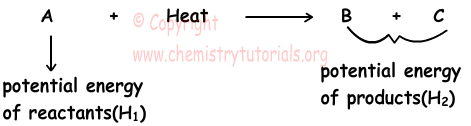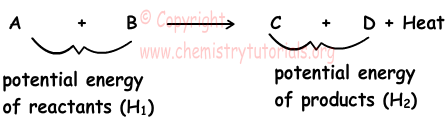EN | ES | DE | NL | RU

## Thermochemistry Cheat Sheet

Thermochemistry Cheat Sheet

Thermochemistry deals with heat (energy) changes in chemical reactions. In chemical reactions heat is released or absorbed. If reaction absorbs heat then we call them endothermic reactions and if reaction release heat we call them exothermic reactions.

Endothermic Reactions:

In endothermic reactions, potential energy of reactants are lower than potential energy of products. To balance this energy difference, heat is given to reaction. Potential energy is shown with H.Exothermic Reactions:

Condensation of gases, combustion reactions are examples of exothermic reactions. In these reactions, potential energies of reactants are higher than potential energies of products. Excess amount of energy is written in right side of reaction to balance energy difference.Enthalpy and Thermochemical Reactions

Physical and chemical changes are done under constant pressure. Gained or lost heat in reactions under constant pressure is called enthalpy change. Enthalpy is the total kinetic and potential energy of particles of matter. It is denoted by letter "H".

If HR is the enthalpy of reactants and HP is the enthalpy of products, change in enthalpy becomes,

∆H=HP-HR

• In exothermic reactions, HR is larger than HP, so enthalpy change becomes negative

HP<HR so; ∆H<0

• Since endothermic reactions absorb heat, HP>HR and enthalpy change becomes positive.

HP>HR so; ∆H>0

• Enthalpy change depends on temperature and pressure. Thus, you should compare enthalpy changes of reactions under same temperature and pressure.
• Enthalpy change under 1 atm pressure and 25 0C temperature is called standard enthalpy change.

Reactions showing both changes of matters and energy are called thermochemical reactions. Examples of thermochemical reactions;

• Exothermic reaction;

C(s) +O2(g) → CO2(g) ; ∆H=-94 kcal

• Endothermic reaction;

2H2O(g) → 2H2(g) + O2(g) ; ∆H=116 kcal

Properties of Thermochemical Reactions

• Coefficients in front of each element shows number of moles of matters and given ∆H value shows heat released or absorbed by reaction balanced with these numbers.
• If you multiply reaction with number "n" than you must multiply ∆H value also with "n".
• If direction of thermochemical reaction is changed, then sign of ∆H is also changed.
• Since ∆H depends on states of matters, you must write states of matters in thermochemical reactions.

Hess' law states that, you can sum one more than one reactions to form new reaction. While doing this, you apply same changes also on enthalpy changes of used reactions.

1) Standard Molar Enthalpy of Formation:

Enthalpy change of formation of 1 mole compound from its elements is called standard molar enthalpy of formation and expressed in kcal/mol or kjoule/mol. Be careful when writing formation reactions an pay attention on following suggestions;

• Reaction must be written for 1 mole compound
• Compound must be formed by elements
• Compound must be formed stable elements

2) Standard Enthalpy of Decomposition:

Enthalpy change of decomposition of  1 mole compound into its elements is called standard molar enthalpy of decomposition.

Example:

H2O(l) → H2(g) + 1/2 O2(g) ; ∆H=68 kcal

3) Standard Enthalpy of Combustion:

It is the heat released from the reaction of one mole element with O2(g).

Example:

CH4(g) + 2O2(g) → CO2(g) + 2H2O(l) ; ∆H=-212 kcal

4) Standard Enthalpy of Neutralization Reaction:

It is the enthalpy change of neutralization of 1 mol acid and one mol base. These reactions are exothermic reactions.

Acid + Base → Salt + Water + Heat

Example:

H+ + OH- → H2O + 13,5 kcal

Forming chemical bond atoms become more stable and their energies decrease and this energy is released outside. While breaking this bond same amount of energy is required. Energy released during formation of one mol bond and required for breaking one mole bond is called bond energy.

Reactants → Products ; ∆H=?

∆H=∑(Bond Energies)Reactants-∑(Bond Energies)Products

Where ∑ shows sum of given quantities.

In a reaction If;

• (Sum of bond energies of reactants) > (Sum of bond energies of products) then, ∆H > 0, in other words reaction is endothermic. Some part of energy required to break bonds of reactants is taken from energy released from formation of bonds of products and some part of it is taken from outside.
• (Sum of bond energies of reactants) < (Sum of bond energies of products) then, ∆H < 0, in other words reaction is exothermic. Thus, some part of the energy released from forming new bonds in products is used for breaking bond in reactants and some part of energy is released outside.

Most of enthalpy change can be measured experimentally. This process is called " measuring heat transfer " calorimetry. Calorimeters are devices used in measuring heat flow. In calorimeters;

Heat Absorbed = Heat Released

Heat flow in calorimeter is calculated with following formula;

Q=mcal.ccal.∆T + mwater. cwater.∆T

Where;

mcal= mass of calorimeter, in g.

ccal=specific heat capacity of calorimeter

mwater= mass of water in g.

cwater= specific heat capacity of water

Enthalpy of the reactions depends on;

• Quantity of matter
• Physical state of matter
• Pressure
• Temperature
• Types of matter

Thermochemistry Exams and  Problem Solutions

The Original Author: